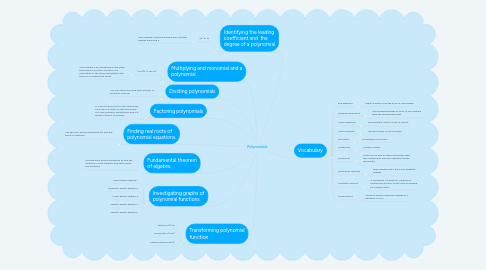# PolynomialsJohn Moshman
Get Started. It's FreePolynomials## 1. Vocabulary

### 1.1. End behavior

1.1.1. What is going on at the ends of each graph.

1.2.1. The numbers written in front of the variable with the largest exponent.

### 1.3. Local maximum

1.3.1. The greatest value in a set of points.

### 1.4. Local minimum

1.4.1. The least value in set of points.

### 1.5. Monomial

1.5.1. Consisting of one term

### 1.6. Multiplicity

1.6.1. A large number

### 1.7. Polynomial

1.7.1. is the sum of one or more monomials with real coefficients and non negative integer exponents.

### 1.8. Polynomial function

1.8.1. real numbers and n are a non negative integer.

### 1.9. Synthetic division

1.9.1. A shorthand, or shortcut, method of polynomial division in the case of dividing by a linear factor.

### 1.10. Turning point

1.10.1. a time at which a decisive change in a situation occurs

## 8. Identifying the leading coefficient and the degree of a polynomial

### 8.1. 5x^3+15

8.1.1. The Leading coefficient would be 5 and the degree would be 3

## 9. Multiplying and monomial and a polynomial

### 9.1. (x+2)(x^2+3x+5)

9.1.1. You multiply x by everything in the other parenthesis and then multiply 2 by everything in the other parenthesis and then you combine like terms.